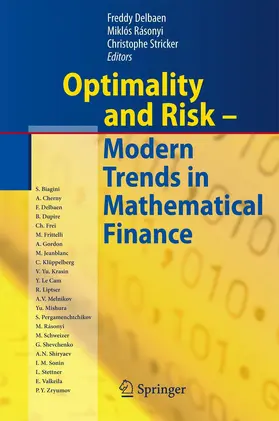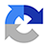# Delbaen / Rásonyi / StrickerOptimality and Risk - Modern Trends in Mathematical Finance

The Kabanov Festschrift

2010, 266 Seiten, Gebunden, Book, Format (B × H): 167 mm x 242 mm, Gewicht: 1280 g
ISBN: 978-3-642-02607-2
Verlag: Springer

## Delbaen / Rásonyi / StrickerOptimality and Risk - Modern Trends in Mathematical Finance

Problems of stochastic optimization and various mathematical aspects of risk are the main themes of this contributed volume. The readers learn about the recent results and techniques of optimal investment, risk measures and derivative pricing. There are also papers touching upon credit risk, martingale theory and limit theorems.

Forefront researchers in probability and financial mathematics have contributed to this volume paying tribute to Yuri Kabanov, an eminent researcher in probability and mathematical finance, on the occasion of his 60th birthday. The volume gives a fair overview of these topics and the current approaches.

Research

## Weitere Infos & Material

On the Extension of the Namioka-Klee Theorem and on the Fatou Property for Risk Measures.- On Certain Distributions Associated with the Range of Martingales.- Differentiability Properties of Utility Functions.- Exponential Utility Indifference Valuation in a General Semimartingale Model.- The Expected Number of Intersections of a Four Valued Bounded Martingale with any Level May be Infinite.- Immersion Property and Credit Risk Modelling.- Optimal Consumption and Investment with Bounded Downside Risk for Power Utility Functions.- On Comparison Theorem and its Applications to Finance.- Examples of FCLT in Random Environment.- The Optimal Time to Exchange one Asset for Another on Finite Interval.- Arbitrage Under Transaction Costs Revisited.- On the Linear and Nonlinear Generalized Bayesian Disorder Problem (Discrete Time Case).- Long Time Growth Optimal Portfolio with Transaction Costs.- On the Approximation of Geometric Fractional Brownian Motion.

## Ihre Fragen, Wünsche oder Anmerkungen

Ihre Nachricht*
Wie möchten Sie kontaktiert werden?
Anrede*
Titel
Vorname*
Nachname*